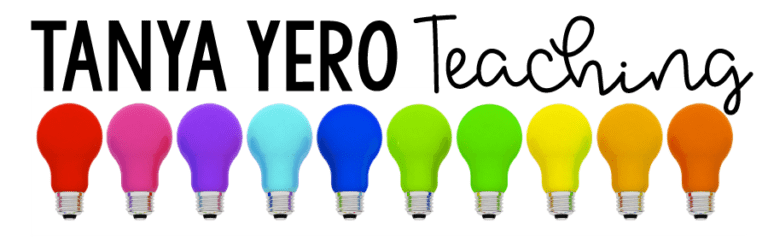Sale!

# 2nd Grade Guided Math YEARLONG BUNDLE Differentiated Work EASY PREP

\$35.00 \$28.00

Total Pages: 400
File Size: 15 MB

## Description

Take the prep work out of guided math rotations and make life easier for yourself. POWER Math Reference Mats tailor instruction for your students with NO PREP on your behalf! Differentiate math with ease!

What are Guided Math Reference Mats?

POWER Math Reference Mats are designed to assist teachers during math rotations. Housed with specific target skills for each level in your class, POWER Math Reference Mats come with various problems for you to give to your students when working in small groups. Group A is for students who are in need of remediation, Group B is for students working on grade level, and Group C is for students who need enrichment. Each mat comes with target skills that are designed to match the needs of each student level in your class. There are questions/problems for each target skill which can be given to your students. Power Math Reference Mats require little materials: whiteboards, expo markers, and erasers or paper and pencils.

~~~~~~~~~~~~~~~~~~~~~~~~~~~~~~~~~~~~~~~~~~~~~~~~~~~~
Standards & Topics Covered

Algebraic Thinking:
➥ 2.OA.1 – Represent and solve addition and subtraction word problems, within 100
➥ 2.OA.2 – Demonstrate fluency with addition and subtraction, within 20, using mental strategies
➥ 2.OA.3 – Determine whether a group of objects, within 20, has an odd or even number of members
➥ 2.OA.4 – Use addition to find the total number of objects arranged in rectangular arrays with up to 5 rows and up to 5 columns; write an equation to express the total as a sum of equal addends.

Geometry:
➥ 2.G.1 – Recognize and draw polygons
➥ 2.G.2 – Partition a rectangle into rows and columns
➥ 2.G.3 – Partition circles and rectangles into two, three, or four equal shares.

Numbers and Operations in Base Ten:
➥ 2.NBT.1 – Understand that the three digits of a three-digit number represent amounts of hundreds, tens, and ones.
➥ 2.NBT.2 – Count within 1,000; skip-count by 5s, 10s, and 100s.
➥ 2.NBT.3 – Read and write numbers, within 1,000, using base-ten numerals, number names, and expanded form.
➥ 2.NBT.4 – Compare two three-digit numbers based on the value of the hundreds, tens, and ones digits.
➥ 2.NBT.5 – Demonstrate fluency with addition and subtraction, within 100.
➥ 2.NBT.6 – Add up to three two-digit numbers using strategies based on place value and properties of operations.
➥ 2.NBT.7 – Add and subtract, within 1,000, relating the strategy to a written method.
➥ 2.NBT.8 – Mentally add 10 or 100 to a given number 100–900, and mentally subtract 10 or 100 from a given number 100–900.

Measurement and Data Topics:
➥ 2.MD.1 – Measure the length of an object in standard units
➥ 2.MD.2 – Measure the length of an object twice, using length units of different lengths for the two measurements
➥ 2.MD.3 – Estimate lengths in using standard units of inches, feet, yards, centimeters, and meters
➥ 2.MD.4 – Measure to determine how much longer one object is than another, expressing the length difference in terms of a standard length unit
➥ 2.MD.5 – Use addition and subtraction, within 100, to solve word problems involving lengths that are given in the same units
➥ 2.MD.6 – Represent whole numbers as lengths from 0 on a number line diagram
➥ 2.MD.7 – Tell and write time from analog and digital clocks to the nearest five minutes, using a.m. and p.m.
➥ 2.MD.8 – Word problems with money
➥ 2.MD.9 – Interpreting data on a line plot
➥ 2.MD.10 – Organize, represent, and interpret data with up to four categories.